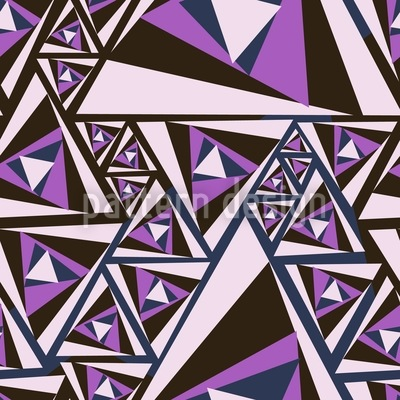••••# Triangle Rotation Seamless Vector Pattern Design

Keywords
Art Deco, Triangle, Twenties, Movement, Geometric, Rotation, Modern, Geometry, 1920s, Geometric Pattern, Geometrical Triangle Rotation design, Triangle Rotation vector, Triangle Rotation pattern, Triangle Rotation all over print, Triangle Rotation illustration, Triangle Rotation ornament, Triangle Rotation pattern design, Triangle Rotation artwork, Triangle Rotation vector art, Triangle Rotation background pattern, Triangle Rotation textile, Triangle Rotation seamless, Triangle Rotation repeating pattern, Triangle Rotation surface
Designer Deborah Eater ID #5664
Description Geometric design with triangle ornaments.
Prices